# 【Python】第七篇：模块化编程及第三方模块的安装

💖少年遥想得山田，请赶在日暮下山💖

# 系列文章目录🍁

### 文章目录

• 系列文章目录🍁
• 🌴前言
• 🌿一、模块化编程
• 1.什么叫模块(Modules)？
• 2.自定义模块
• 3.以主程序的形式执行
• 4.python中的包
• 5.Python中常用的内置模块
• 6.第三方模块的安装及使用
• 安装
• 在线安装方式
• 使用
• 🌴总结

• # 🌴前言

大家好，我是小沐！😃编程路上一个人可能走的更快，但一群人才能走得更远，关注小沐一起学习不迷路！今天分享的是 模块化编程以及第三方模块的安装，快学起来吧，秃头走起——>冲冲冲👊👊👊！！！

# 🌿一、模块化编程

## 1.什么叫模块(Modules)？

模块与函数的关系一个模块中可以包含N多个函数；
在Python中一个扩展名为.py的文件就是一个模块；
模块中含有类、函数和语句
一个程序项目由N个模块组成；

使用模块的好处：
方便其他程序和脚本的导入并使用
避免函数名和变量名冲突（两个模块有相同的变量名不冲突）
提高代码的可维护性
提高代码的可重用性

## 2.自定义模块

创建模块：
新建一个.py文件，名称不要与Python自带的标准模块名称相同

导入模块：
第一种：import 模块名称 [as 别名]
第二种：from 模块名称 import 函数/变量/类

第一种

``````#第一种导入方式
import math
print(id(math))
print(type(math))
print(math)
print(dir(math)) #查看math中的属性方法
print('--------------------------------------')
print(math.pi)
print(math.pow(2,5))
print(math.ceil(9.01))
print(math.floor(9.999))
``````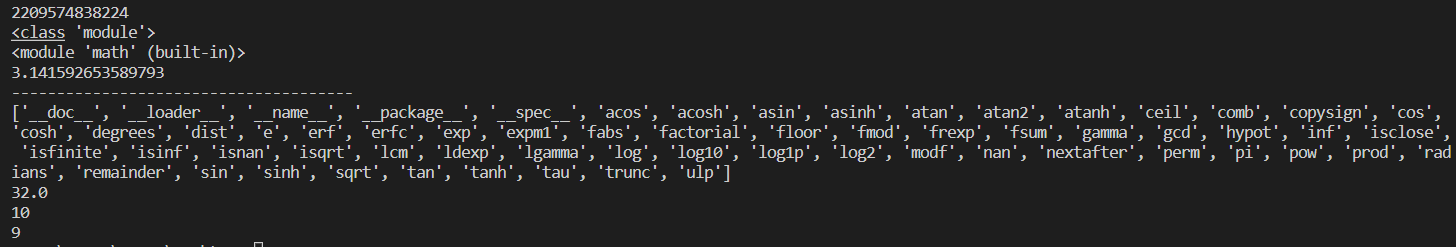第二种

``````#第二种导入方式 只导入模块中指定的一部分
from math import pi
print(pi)
print(pow(2,3))
from math import pow
print(pow(2,3))
``````

同样的，导入自己写的模块也是一样的方法。

## 3.以主程序的形式执行

有时候我们在调用其他模块的内容的时候，由于在其他模块的定义中会输出一些语句，导致在我们的当前模块输出内容时，也会输出调用模块的输出内容，这时候，我们可以在所调用的模块输出前加条件：

``````if __name__=='__main__':
pass
``````

只有这个模块为主程序运行时，才会执行pass

## 4.python中的包

：是一个分层次的目录结构，它将一组功能相近的模块组织在一起。
作用：代码规范、避免模块名称冲突（不同包中不同模块模块名可以相同）
包与目录的区别：
包含__init__.py文件的目录称为包
目录通常不包含__init__.py文件

包的导入：
import 包名.模块名

``````import pagekge.module_A
print(pagekge.moudle_A.a)#调用一个包里的一个模块的一个属性或函数
import pagekge.module_A as mm #取小名
print(mm.a)
``````

总结注意：
使用import开头导入方式只能导入包名或模块名
使用from…import导入方式可以导入包、模块、函数、变量

## 5.Python中常用的内置模块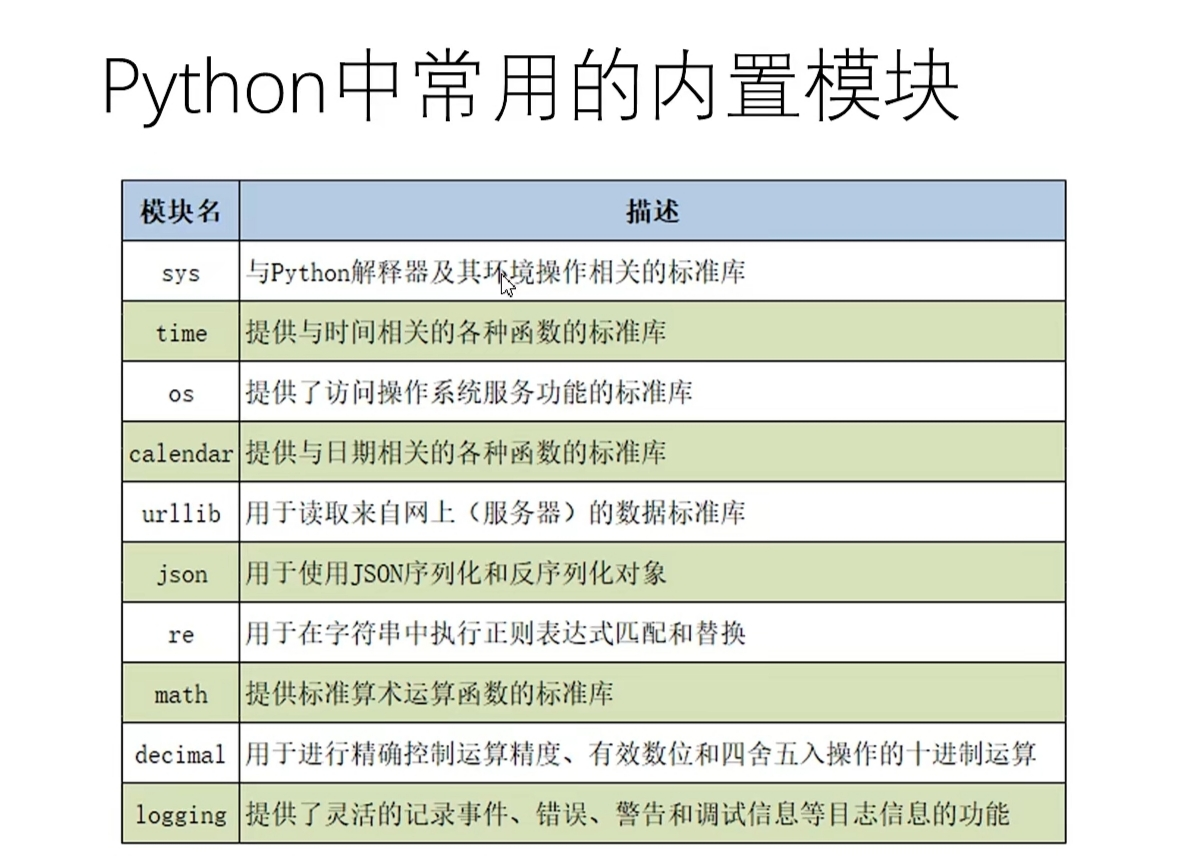``````import sys
print(sys.getsizeof(24))
print(sys.getsizeof(99))
print(sys.getsizeof(True))
print(sys.getsizeof(False))
import time
print(time.time()) #输出秒
print(time.localtime(time.time())) #输出当前时间
import urllib.request  #与爬虫有关的
``````

## 6.第三方模块的安装及使用

Python的强大离不开第三方模块。有很多人写了很多第三方模块实现了很多功能，而我们只需安装就可使用。

### 安装

pip install 模块名

#### 在线安装方式

以安装schedule模块为例：
第一步：windows徽标键+R调出窗口，输入cmd，点击确定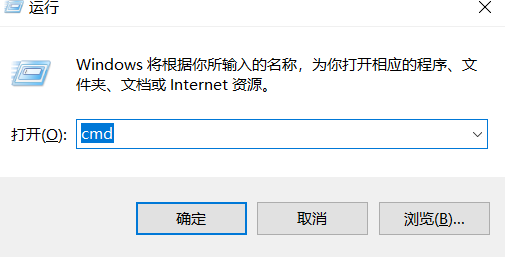第二步：输入pip install+要安装的模块名称，按回车键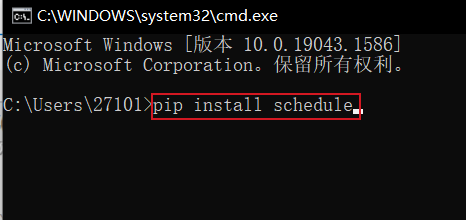第三步：输入python，回车进入python的交互式程序，输入 import +模块名，回车，如果没有报错则安装成功！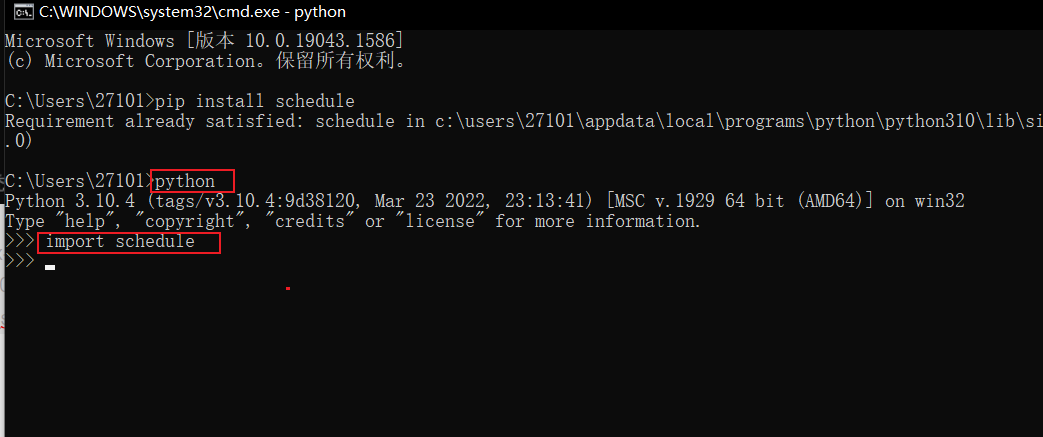### 使用

import 模块名

``````import schedule
import time
def job():
print('666666')

schedule.every(3).seconds.do(job)
while True:
schedule.run_pending()  #检测job的时间到了没有
time.sleep(1)

``````

# 🌴总结

今日分享到此结束👊👊👊，由于笔者还在求学之路上辗转徘徊🏃，水平有限，文章中可能有不对之处，还请各位大佬指正🙏，祝愿每一个热爱编程的人都能实现追求，考研上岸进大厂，熬夜秃头卷中王。最后欢迎关注小沐，学习路上不迷路！😜

来源：小沐想秃头